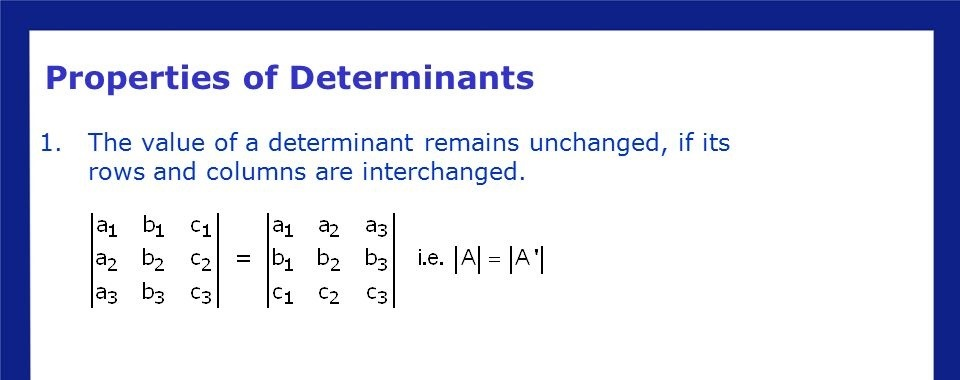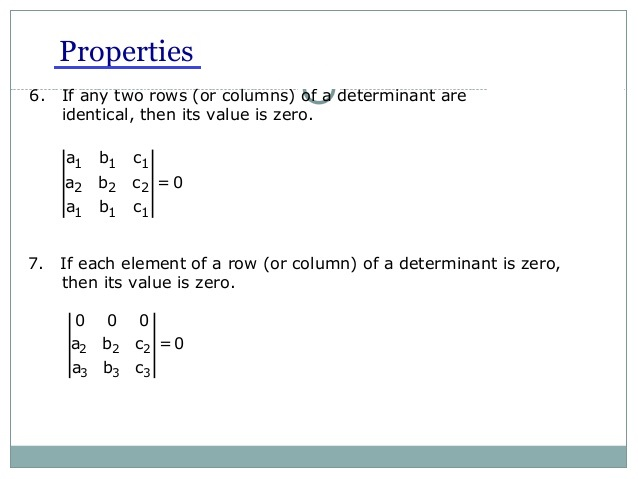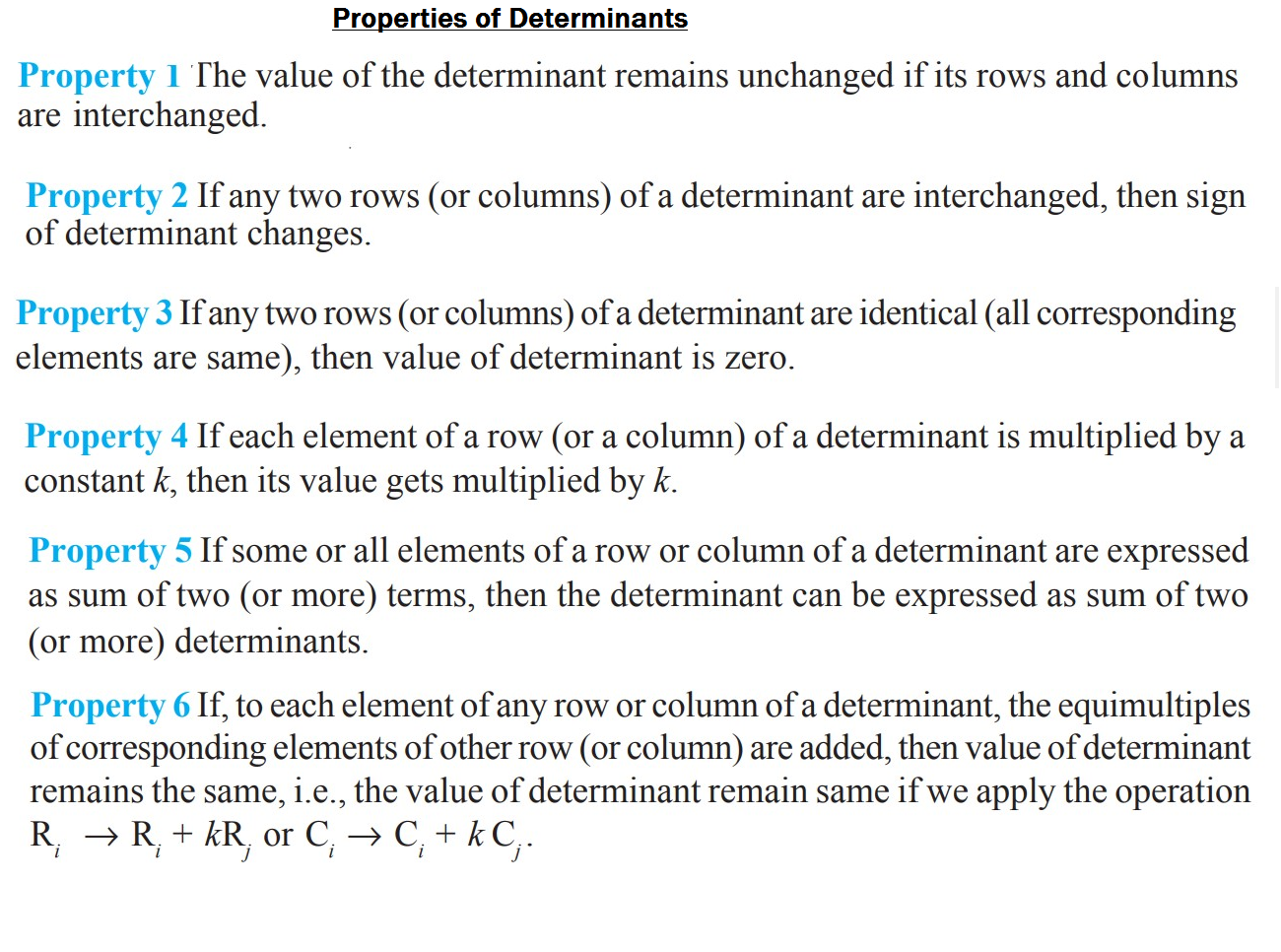Mathematics Properties of Determinants For CBSE-NCERT
Click for Only Video

### Topic covered

star Properties of Determinants

### Property 1 :color{red}{"The value of the determinant remains unchanged if its rows and columns are interchanged."}

Verification : Let Delta = |(a_1,a_2,a_3),(b_1,b_2,b_3),(c_1,c_2,c_3)|

Expanding along first row, we get Delta = a_1|(b_2,b_3),(c_2,c_3)| - a_2|(b_1,b_3),(c_1,c_3)| + a_3|(b_1,b_2),(c_1,c_2)|

=a_1(b_2 c_3– b_3 c_2) - a_2(b_1 c_3– b_3 c_1) + a_3(b_1 c_2 – b_2 c_1)

● By interchanging the rows and columns of Δ, we get the determinant

 Δ_1 = | (a_1 ,b_1 ,c_1), ( a_2,b_2, c_2), ( a_3,b_3,c_3) |

● Expanding Δ_1 along first column, we get

Δ_1 = a_1 (b_2 c_3 – c_2 b_3) – a_2 (b_1 c_3 – b_3 c_1) + a_3 (b_1 c_2 – b_2 c_1)

Hence Δ = Δ_1

color{blue}{"Remark :"} If A is a square matrix, then color{orange}{det (A) = det (A′),} where A′ = transpose of A.

color{blue}{"Note :"} If R_i = i^(th) row and C_i = i^(th) column, then for interchange of row and columns, we will symbolically write C_i↔ R_i
Q 3154180954Verify Property 1 for Δ = | ( 2,-3, 5), ( 6,0,4), ( 1,5, -7) |
Class 12 Chapter 4 Example 6Solution:

Expanding the determinant along first row, we have

 Δ = 2 | ( 0 ,4) , ( 5,-7) | - (-3) | (6,4), ( 1,-7) | + 5 | ( 6,0 ), (1,5) |

= 2 (0 – 20) + 3 (– 42 – 4) + 5 (30 – 0)
= – 40 – 138 + 150 = – 28
By interchanging rows and columns, we get

Δ_1 = | (2,6,1), (-3,0,5), (5,4 , -7) | (Expanding along first column)

=2 | ( 0 ,5 ), (4,-7) | - (-3) | ( 6,1), (4 , -7) | + 5 | ( 6,1), ( 0 ,5) |

= 2 (0 – 20) + 3 (– 42 – 4) + 5 (30 – 0)
= – 40 – 138 + 150 = – 28
Clearly Δ = Δ_1

Hence, Property 1 is verified.

### Property 2 :color{red}{"If any two rows (or columns) of a determinant are interchanged,"}
color{red}{"then sign of determinant changes. "}

"Verification :" Let Δ =  | (a_1, a_2, a_3 ), ( b_1, b_2 , b_3 ), ( c_1, c_2, c_3) |

Expanding along first row, we get

Δ = a_1 (b_2 c_3 – b_3 c_2) – a_2 (b_1 c_3 – b_3 c_1) + a_3 (b_1 c_2 – b_2 c_1)

=> Interchanging first and third rows, the new determinant obtained is given by

Δ_1 = | (c_1, c_2, c_3 ), ( b_1, b_2 , b_3 ), ( a_1, a_2 , a_3) |

=> Expanding along third row, we get

Δ_1 = a_1 (c_2 b_3 – b_2 c_3) – a_2 (c_1 b_3 – c_3 b_1) + a_3 (b_2 c_1 – b_1 c_2)

= – [a_1 (b_2 c_3 – b_3 c_2) – a_2 (b_1 c_3 – b_3 c_1) + a_3 (b_1 c_2 – b_2 c_1)]

Clearly Δ_1 = – Δ

"Similarly, we can verify the result by interchanging any two columns."

color{orange}{" We can denote the interchange of rows by"\ \ R_i ↔ R_j \ \ "and interchange of
columns by" C_i ↔ C_j}.

Q 3184180957Verify Property 2 for Δ = | (2,-3,5), ( 6,0,4), ( 1,5, -7) |
Class 12 Chapter 4 Example 7Solution:

Δ = | (2,-3,5), ( 6,0,4), ( 1,5, -7) | = -28 (See Example 6)

Interchanging rows R_2 and R_3 i.e., R_2 ↔ R_3, we have

Δ_1 = | ( 2,-3 ,5), ( 1,5, -7) , ( 6,0,4) |

Expanding the determinant Δ_1 along first row, we have

Δ_1 = 2 | ( 5,-7), (0,4) | - ( -3) | (1,-7), (6,4) | + 5 | ( 1,5), (6 ,0) |

= 2 (20 – 0) + 3 (4 + 42) + 5 (0 – 30)
= 40 + 138 – 150 = 28

Clearly Δ_1 = – Δ

Hence, Property 2 is verified

### Property 3 :color{red}{" If any two rows (or columns) of a determinant are identical "}
color{red}{"all corresponding elements are same), then value of determinant is zero."}

color{blue}{"Proof"} If we interchange the identical rows (or columns) of the determinant Δ, then Δ
does not change. However, by Property 2, it follows that Δ has changed its sign

Therefore Δ = – Δ

or Δ = 0

Q 3154191054Evaluate Δ = | ( 3,2,3), ( 2,2,3), ( 3,2,3) |
Class 12 Chapter 4 Example 8Solution:

Expanding along first row, we get

Δ = 3 (6 – 6) – 2 (6 – 9) + 3 (4 – 6)
= 0 – 2 (–3) + 3 (–2) = 6 – 6 = 0
Here R_1 and R_3 are identical.

### Property 4 :color{red}{" If each element of a row (or a column) of a determinant is"}
color{green}{"multiplied by a constant" k, "then its value gets multiplied by" k}

"Verification :" Let Δ = | (a_1, b_1, c_1), ( a_2,b_2, c_2), (a_3,b_3,c_3) |

and Δ_1 be the determinant obtained by multiplying the elements of the first row by k.

Then

Δ_1 = | (k a_1, k b_1, k c_1 ), ( a_2, b_2, c_2 ), ( a_3, b_3, c_3) |

=> Expanding along first row, we get

Δ_1 = k a_1 (b_2 c_3 – b_3 c_2) – k b_1 (a_2 c_3 – c_2 a_3) + k c_1 (a_2 b_3 – b_2 a_3)

= k [ a_1 (b_2 c_3 – b_3 c_2) – b_1 (a_2 c_3 – c_2 a_3) + c_1 (a_2 b_3 – b_2 a_3)]

= k Δ

color{orange}{ | (k a_1, k b_1, k c_1 ), ( a_2, b_2, c_2), ( a_3, b_3, c_3) | = k | (a_1, b_1, c_1), ( a_2,b_2 ,c_2), (a_3, b_3, c_3) |}

color{blue}{"Remarks :"}

(i) By this property, we can take out any common factor from any one row or any one column of a given determinant.
(ii) If corresponding elements of any two rows (or columns) of a determinant are proportional (in the same ratio), then its value is zero. For example
Δ = | (a_1, a_2, a_3 ), (b_1, b_2, b_3 ), ( k a_1, k a_2, k a_3) |=0  (rows R_1 and R_2 are proportional)
Q 3104191058Evaluate  | (102, 18 ,36) , (1,3,4), ( 17,3,6) |
Class 12 Chapter 4 Example 9Solution:

Note that  | (102,18 , 36), (1,3, 4 ), (17 ,3,6 ) | = | ( 6 (17) , 6 (3) , 6(6) ), ( 1,3,4), ( 17,3,6) | = 6 | (17 ,3,6 ), ( 1,3,4), ( 17,3,6) | = 0

### Property 5color{red}{"If some or all elements of a row or column of a determinant are expressed as "}
color{red}{"sum of two (or more) terms, then the determinant can be expressed as sum of two (or more) determinants."}

For example,  | (a_1+ lambda_1 , a_2 + lambda_2, a_3 + lambda_3 ), ( b_1, b_2, b_3 ), ( c_1, c_2, c_3) |

= | (a_1, a_2, a_3 ), ( b_1, b_2 , b_3), (c_1, c_2 , c_3) | + | ( lambda_1, lambda_2, lambda_3 ) , ( b_1, b_2, b_3), (c_!, c_2, c_3) |

Verification L.H.S. = | (a_1 + lambda_1 , a_2 +lambda_2 , a_3+lambda_3 ), ( b_1, b_2, b_3), ( c_1, c_2, c_3) |

=> Expanding the determinants along the first row, we get

Δ = (a_1 + λ_1) (b_2 c_3 – c_2 b_3) – (a_2 + λ_2) (b_1 c_3 – b_3 c_1)

+ (a_3 + λ_3) (b_1 c_2 – b_2 c_1)

= a_1 (b_2 c_3 – c_2 b_3) – a_2 (b_1 c_3 – b_3 c_1) + a_3 (b_1 c_2 – b_2 c_1)

+ λ_1 (b_2 c_3 – c_2 b_3) – λ_2 (b_1 c_3 – b_3 c_1) + λ_3 (b_1 c_2 – b_2 c_1)
(by rearranging terms)

= | (a_1, a_2, a_3), ( b_1, b_2, b_3), ( c_1, c_2, c_3) | + | (λ_1 , λ_2, λ_3 ), ( b_1 ,b_2, b_3 ), ( c_1, c_2, c_3) | = R.H.S.

=> Similarly, we may verify Property 5 for other rows or columns.
Q 3124291151Show that  | (a,b,c ), ( a+2x , b +2y , c +2z), ( x,y,z ) | = 0
Class 12 Chapter 4 Example 10Solution:

We have | ( a,b,c), ( a+2x , b +2y ,c+ 2z), ( x,y,z) | = | ( a,b,c ), ( a,b,c), ( x,y,z) | + | (a,b,c), ( 2x +2y +2z), ( x,y,z ) |

= 0 +0 += 0

### Property 6color{green} ✍️ If to each element of any row or column of a determinant, the equimultiples of corresponding elements of other row (or column) are added, then value of determinant remains the same, i.e., the value of determinant remain same if we apply the operation

color{red}{R_i → R_i + k R_j or C_i → C_i + k C_j} .

"Verification : " Let  Δ = | (a_1, a_2, a_3), ( b_1, b_2, b_3), (c_1, c_2, c_3) |
and  Δ_1 = | (a_1+k c_1 , a_2 + kc_2 , a_3 + k c_3), ( b_1, b_2, b_3 ), ( c_1, c_2, c_3) | ,

=> where Δ_1 is obtained by the operation R_1 → R_1 + k R_3 .

=> Here, we have multiplied the elements of the third row (R_3) by a constant k and added them to the corresponding elements of the first row (R_1).

Now, again

 Δ_1 = | (a_1 , a_2, a_3), ( b_1, b_2, b_3), ( c_!, c_2, c_3) | + | (k c_1, k c_2, k c_3), (b_1, b_2, b_3), ( c_1, c_2, c_3) |  (Using Property 5)

= Δ + 0 (since R_1 and R_3 are proportional)

Hence Δ = Δ_1

Remarks

(i) If Δ_1 is the determinant obtained by applying R_i → k R_i or C_i → k C_i to the determinant Δ, then Δ_1 = kΔ.

(ii) If more than one operation like R_i→ R_i + k R_j is done in one step, care should be taken to see that a row that is affected in one operation should not be used in another operation. A similar remark applies to column operations.
Q 3144291153Prove that  | ( a,a+b , a+b +c ), ( 2a , 3a +2b , 4a +3b +2c ), ( 3a , 6a +3b , 10a + 6b + 3c) | = a^3

Class 12 Chapter 4 Example 11Solution:

Applying operations R_2 → R_2 – 2R_1 and R_3 → R_3 – 3R_1 to the given
determinant Δ, we have

Δ = | (a, a+b , a+b +c ), ( 0,a, a + b ), ( 0 ,3a , 7a +3b) |

Now applying R_3 → R_3 – 3R_2 , we get

Δ = | ( a, a+b , a+b +c ), ( 0,a, 2a +b), ( 0,0, a) |

Expanding along C_1, we obtain

Δ = a | (a, 2a + b ) , ( 0 ,a) | + 0 + 0

 = a (a^2 -0 ) = a (a^2 ) = a^3
Q 3154291154Without expanding, prove that

Δ = | ( x+y , y+z, z+x ), ( z,x,y), ( 1,1,1) | = 0
Class 12 Chapter 4 Example 12Solution:

Applying R_1 → R_1 + R_2 to Δ, we get

Δ = | ( x+y +z, x+y +z , x+ y + z ), ( z ,x,y), ( 1,1,1) |

Since the elements of R_1 and R_3 are proportional, Δ = 0.
Q 3164291155Evaluate

 Δ = | ( 1,a, bc ), ( 1,b, ca), ( 1,c, ab ) |
Class 12 Chapter 4 Example 13Solution:

Applying R_2 → R_2 – R_1 and R_3 → R_3 – R_1, we get

Δ = | (1,a, bc ), ( 0 , b-a , c (a-b) ) ,( 0 , c-a , b (a-c) ) |

Taking factors (b – a) and (c – a) common from R_2 and R_3, respectively, we get

Δ = (b-a) (c-a) | ( 1,a, bc) , ( 0,1,-c), ( 0,1, -b) |

= (b – a) (c – a) [(– b + c)] (Expanding along first column)
= (a – b) (b – c) (c – a)
Q 3114291159Prove that  | ( b+c ,a,a ), ( b, c+a, b ), ( c,c , a+b ) | = 4 abc
Class 12 Chapter 4 Example 14Solution:

Let Δ = | ( b+c, a,a), ( b, c+a, b), ( c,c, a+b ) |

Applying R_1 → R_1 – R_2 – R_3 to Δ, we get

Δ = | ( 0 , -2c , -2b ), ( b, c+a , b ), ( c,c, a+b ) |

Expanding along R_1, we obtain

 Δ = 0 | ( c+a , b ), ( c, a+b ) | - (-2c) | ( b,b), ( c, a+b) | + (-2b ) | ( b , c+a ), ( c,c) |

= 2 c (a b + b^2 – bc) – 2 b (b c – c^2 – ac)

= 2 a b c + 2 cb^2 – 2 bc^2 – 2 b^2 c + 2 bc^2 + 2 abc

= 4 abc
Q 3114491350If x, y, z are different and  Δ = | (x,x^2 , 1+x^3 ), ( y , y^2 , 1+ y^3 ), ( z , z^2 , 1+z^3) | = 0 , then

show that 1 + xyz = 0
Class 12 Chapter 4 Example 15Solution:

We have

 Δ = | ( x, x^2 , 1+x^3 ), ( y, y^2 , 1+ y^3 ), ( z, z^2 ,1+z^3 ) |

= | ( x, x^2 ,1 ), ( y , y^2 ,1), ( z, z^2 ,1) | + | ( x, x^2 , x^3 ), ( y , y^2 , y^3 ), (z, z^2 , z^3) |  (Using Property 5)

= (-1)^2 | (1, x, x^2 ), ( 1, y , y^2 ), (1,z, z^2 ) | + xyz | ( 1,x, x^2 ), ( 1,y, y^2 ), ( 1,z, z^2) | (Using C_3↔C_2 and then C_1 ↔ C_2 )

 = | (1,x,x^2 ), (1,y, y^2 ) , ( 1,z, z^2 ) | (1+ xyz)

= (1+ xyz) | (1,x, x^2 ), ( 0 , y-x , y^2 - x^2 ), ( 0, z-x , z^2 - x^2) | (Using R_2→R_2–R_1 and R_3 → R_3–R_1)

Taking out common factor (y – x) from R_2 and (z – x) from R_3, we get

 Δ = (1+xyz) (y-x) (z-x) | ( 1,x, x^2 ) , ( 0,1, y+x), ( 0,1, z+x) |

= (1 + xyz) (y – x) (z – x) (z – y) (on expanding along C_1)
Since Δ = 0 and x, y, z are all different, i.e., x – y ≠ 0, y – z ≠ 0, z – x ≠ 0, we get
1 + xyz = 0
Q 3184491357Show that

 | (1+a ,1,1 ), ( 1,1+b ,1 ), ( 1,1, 1+c) | = abc (1+ 1/a +1/b +1/c) = abc + bc + ca +ab

Class 12 Chapter 4 Example 16Solution:

Taking out factors a,b,c common from R_1, R_2 and R_3 , we get

L.H.S. = abc | (1/a +1, 1/a ,1/a), ( 1/b , 1/b+1, 1/b ), ( 1/c , 1/c , 1/c +1 ) |

Applying R_1→ R_1 + R_2 + R_3, we have

 Δ = abc | (1 +1/a +1/b +1/c , 1 +1/a +1/b +1/c ,1+1/a + 1/b +1/c ), (1/b , 1/b+1 ,1/b), ( 1/c , 1/c ,1/c+1) |

= abc (1 + 1/a +1/b +1/c) | (1,1,1), (1/b , 1/b+1 , 1/b), ( 1/c ,1/c ,1/c+1) |

Now applying C_2 → C_2 – C_1, C_3 → C_3 – C_1, we get

 Δ = abc (1 +1/a +1/b +1/c) | (1,0,0 ), (1/b , 1, 0 ), ( 1/c , 0 ,1 ) |

= abc (1 + 1/a +1/b +1/c) [ 1 (1-0 ) ]

= abc (1+ 1/a + 1/b +1/c) = abc + bc + ca + ab =RHS

### Properties of determinants